# Normalization in a Database with Example Tables

November 6, 2020

Here we discuss the normalization in a database with example tables. Normalization a process that minimizes the redundancy in database.

Normalization was developed by EF code in 1972. The main objective of normalization is to develop a logical data model for a relational database system is to create an accurate representation of data, its relationship, and constraints.

To achieve this objective, we must identify a suitable set of relations. Normalization is often performed as a series of tests on a relation to determine whether it satisfies or violates the recruitments of a given normal form. Based on functional dependencies among the attributes of a relation.

A relation can be normalized to a specific form to prevent the possible occurrence of update anomalies.

One goal of the relational schema design is to minimize the storage space that the base relation ( files )  occupies. Grouping attributes into relation schemas as significant effect storage space. Storing the same information redundantly, that is, in more than one place within a database, can lead following anomalies:

• Update Anomalies: If one copy of a repeated data is updated, an inconsistency is created unless all copies are similarly updated.
• Insertion Anomalies: It may not be possible to store certain information unless some other, unrelated information is stored as well.
• Deletion Anomalies: It may not be possible to delete certain information without losing some other, unrelated, information as well.

Normalization is a method to remove all these anomalies and bring the database to a consistent state.

### The Process of Normalization

Normalization is a formal technique for analyzing a relation based on its primary key and the functional dependencies between the attributes of that relation. It is often executed as a series of steps. Each step corresponds to a specific normal form which has non-properties.

As normalization proceeds, the relations become progressively more restricted ( stronger ) in format and also less vulnerable to update anomalies. The principles of normalization are described in a series of progressively stricter ‘normal forms’.

## Normalization in a Database with Example Tables

Now let’s discuss about the normalization in database with example tables and figures. So that you can understand the idea and rules easily.

### First Normal Form (1NF)

A table is in first normal form if it contains no repeating groups. It means A relation in which the intersection of each row and column and contains one and only one value is said to be in first normal form.

That is, it is stated that the domain of an attribute must include only atomic values. A domain is atomic if elements of the domain are considered to be indivisible units.

Example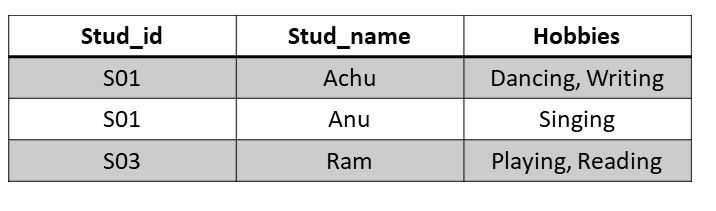In this relation R, attribute hobbies contain more than one atomic value. It violates the rules of 1FN. Thus, by reconstructing the above relation as below to convert it to 1NF, each attribute must contain only a single value from its pre-defined domain.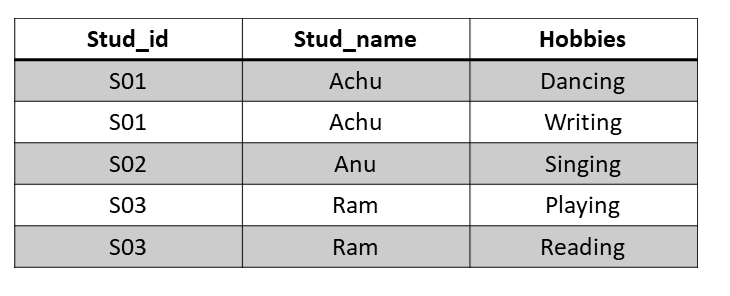### Second Normal Form (2NF)

The second normal form(2NF) is based on the concept of fully functional dependency. A relation  R is in 2NF if it is in 1NF and every non-prime attribute(non-prime attributes is not a part of the primary key) of R is fully dependent on the primary key.

That is, if X->A holds, then there should not be any proper subset Y of X, for which Y->A also holds true.

Example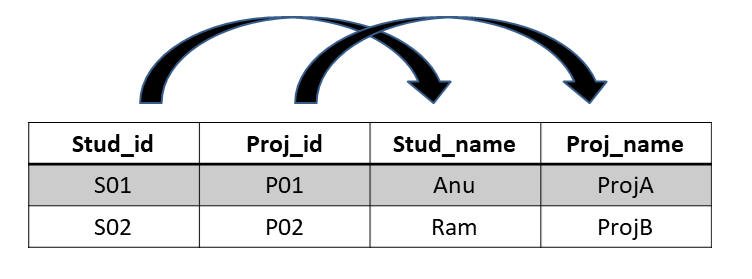We see here in this relation that the prime key attributes are Stud_id and Proj_id. According to rule, non-key attributes, i.e. Stud_name and Proj_name must be dependent upon both and not on any of the prime key attributes individually.

But we find that Stud_name can be identified by Stud_id and Proj_name can be identified by Proj_id independently. This is called Partial dependency, which is not allowed in 2NF.

We broke the relation in two as depicted in the figure. So there exists no partial dependency.

### Third Normal Form (3NF)

The third normal form ( 3NF ) is based on the concept of transitive dependency. A functional dependency X ->Y in a relation R is a transitive dependency if there is a set of attributes Z that is neither a candidate key nor a subset of any key od R, and both X->Z and Z->Y hold.

According to Codd’s original definition, a relation schema R is in 3NF if it satisfies 2NF non-key attributes of R is transitively dependent on the primary key. For any non-trivial functional dependency X->A, then either – X is a super key or A is a prime attribute.

Example

We find that in the above relation, Stud_id is the key and only prime attribute. We find that City can be identified by Stud_id as well as ZIP itself. Neither ZIP is a superkey nor is City a prime attribute. Additionally, Stud_id->ZIP->City, so there exists transitive dependency.

To bring this relation into third normal form, we break the relation into two relations as follows: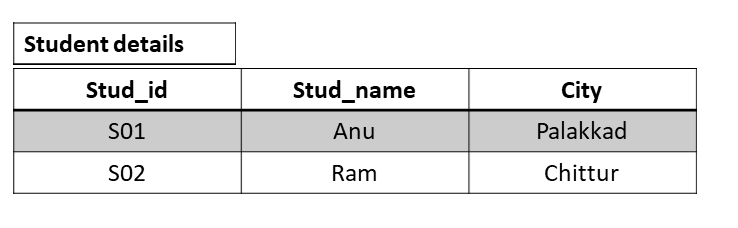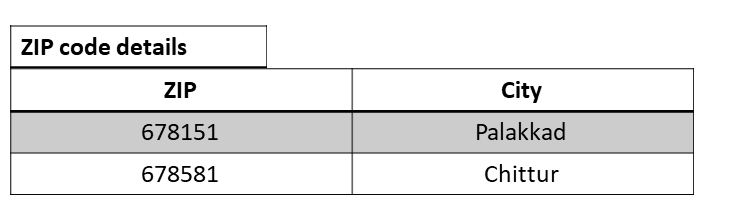### Boyce-Codd Normal Form (BCNF)

Boyce-Codd normal form ( BCNF ) was proposed as a simpler form of 3NF, but it was found to be stricter than 3NF because every relation in BCNF is also in 3NF; a relation in 3NF is not necessarily in BCNF. It is stated that for ->any non-trivial functional dependency, X->A, X must be a super-key.

Example

From the same example as in 3NF, Stud_id is the super-key in the relation Student details and ZIP is the super-key in the relation Zipcode details. So, Stud_id->Stud_name, ZIP and ZIP->City, which confirms that both the relations are in BCNF.

### Fourth Normal Form (4NF)

A relation will be in 4NF if it is in Boyce-Codd normal form and has no multi-valued dependency. For a dependency A->B, if for a single value of A, multiple values of B exists, then the relation will be a multi-valued dependency.

When the existence of one or more rows in a table implies one or more other rows in the same table, then the multi-valued dependencies occur.

Example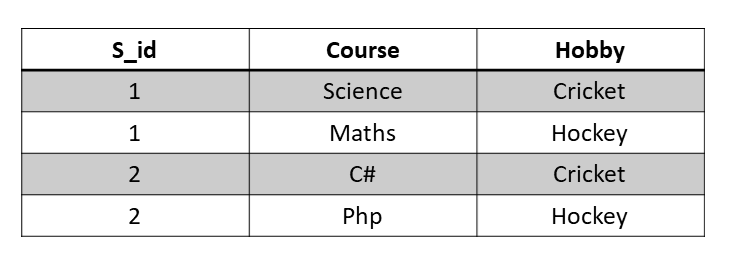In the above relation above, student with S_id has opted for two courses, Science and Maths, and has two hobbies, Cricket and Hockey. So there is multi-value dependency occurred. To make it in 4NF, we can decompose the table into two tables.

Now, this relation satisfies the 4NF, the functionally dependent columns are moved in a separate table and the multi-valued dependent columns are moved to separate tables.

### Fifth Normal Form (5NF)

A relation is in 5NF if it is in 4NF and not contains any join dependency and joining should be lossless. A joint dependency (JD ) can be said to exist if the join of R1 and R2 over C is equal to R. Where, R1 and R2 are the decompositions R1(A, B, C), and R2(C, D) of a relation R(A, B, C, D). 5NF is also known as Project-Join normal form(PJNF).

ExampleIn the above table, John takes both Maths and Physics for class V. Suppose we add a new class as IX but do not know about the subjects and teachers. But all three columns together act as a primary key, so, we leave the other two columns blank. So to make it is in 5NF, we can decompose it into three relations as follows: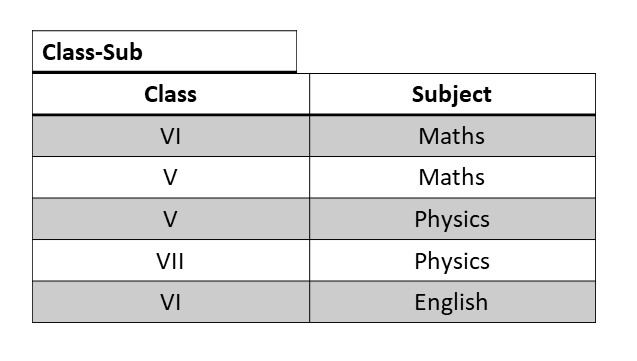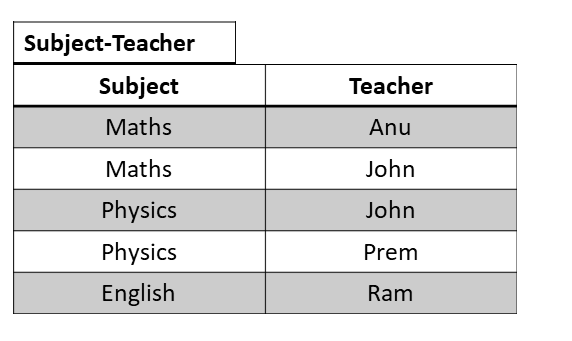Tagged in: ,